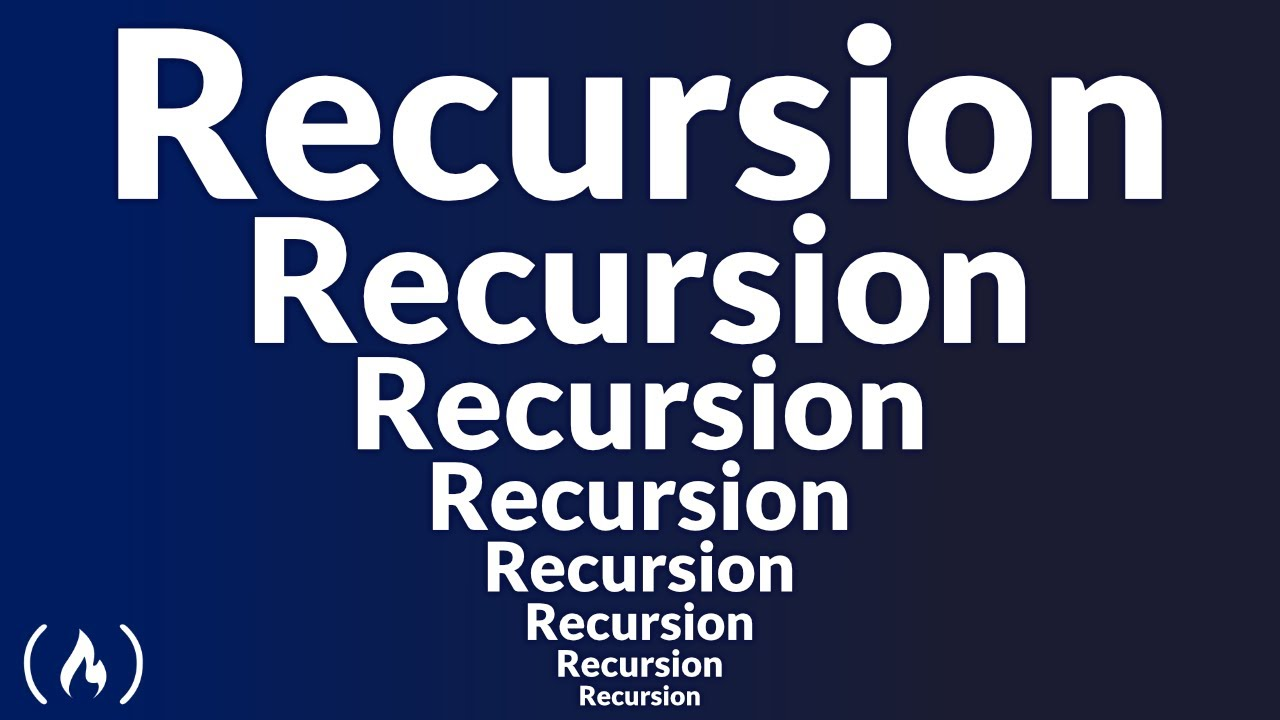# Recursion in Programming - Full CourseRecursion is a powerful technique that helps us bridge the gap between complex problems being solved with elegant code. Within this course, we will break down what recursion is, why you would and wouldn’t want to use it and look at a variety of examples for how it can be used.

Recursion is a powerful technique that helps us bridge the gap between complex problems being solved with elegant code. Within this course, we will break down what recursion is, why you would and wouldn’t want to use it and look at a variety of examples for how it can be used.

We’ll break down recursion with all sorts of data-structures, animations, debugging and call-stack analysis to get a deeper understanding to these principles.

The code is written in Java, but the principles are agnostic to any language.

⭐️ Course Contents ⭐️
⌨️ (0:00:00) Introduction
⌨️ (0:00:42) What Is Recursion?
⌨️ (0:00:50) Explaining Recursion via ATM Analogy
⌨️ (0:04:56) Explaining Recursion via Essay Revision Analogy
⌨️ (0:06:22) Summarizing What Recursion Is
⌨️ (0:07:15) Why & Why Not Recursion
⌨️ (0:10:57) Understanding The Call Stack
⌨️ (0:11:32) Call Stack Analogy
⌨️ (0:16:35) Recursion With Strings Introduction
⌨️ (0:16:50) String Reversal Explanation
⌨️ (0:20:47) String Reversal Call Stack Animation
⌨️ (0:22:59) Palindrome Explanation
⌨️ (0:25:46) Palindrome Call Stack Animation
⌨️ (0:27:46) Recursion With Numbers
⌨️ (0:28:02) Decimal To Binary Explanation
⌨️ (0:31:12) Decimal To Binary Code & Debug
⌨️ (0:34:33) Sum of Natural Numbers Explanation
⌨️ (0:36:17) Sum of Natural Numbers Code & Debug
⌨️ (0:38:51) Divide & Conquer Algorithms
⌨️ (0:39:27) Binary Search Animation & Explanation
⌨️ (0:43:50) Fibonacci Explanation
⌨️ (0:45:54) Fibonacci Animation
⌨️ (0:49:07) Merge Sort Explanation & Animation
⌨️ (0:53:21) Merge Sort Code & Debug
⌨️ (1:09:49) Linked List Reversal Animation
⌨️ (1:14:48) Linked List Code & Debug
⌨️ (1:19:52) Merge Two Sorted Linked Lists Animation
⌨️ (1:25:53) Merge Two Sorted Linked Lists Code & Debug
⌨️ (1:29:02) Trees
⌨️ (1:29:14) Insert Value Into Binary Search Tree Animation
⌨️ (1:31:32) Insert Value Into Binary Search Tree Code Walkthrough
⌨️ (1:33:43) Insert Value Into Binary Search Tree Call Stack Animation
⌨️ (1:35:11) Print All Leaf Nodes Explanation
⌨️ (1:37:02) Print All Leaf Nodes Code & Debug
⌨️ (1:41:09) Graphs
⌨️ (1:41:21) Depth-First Search Animation
⌨️ (1:42:58) Depth-First Search Code Walkthrough
⌨️ (1:45:38) Recursion Optimizations
⌨️ (1:45:44) Memoization & Caching
⌨️ (1:47:59) Tail-Call Recursion
⌨️ (1:50:48) Conclusion